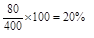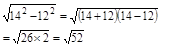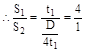# Sample Aptitude Questions of Robert Bosch

1. The average of nine numbers is 50. The average of first five numbers is 54 and the average of last three numbers is 52. What will be the value of sixth number?
1. 24
2. 44
3. 30
4. 34

The sixth number = 9 * 50 – 5 * 54 – 3 * 52 = 450 – 270 – 156 = 24.
2. The average of the first nine integral multiples of 3 is
1. 15
2. 18
3. 21
4. 12

Required average = 3(1+2+3+...+9)/9 = (9 * 10)/(2*3) = 15
1. An article is sold for Rs. 300 at a profit of 20%. Had it been sold for Rs. 235, the loss percentage would have been
1. 5
2. 6
3. 16
4. 3

C.P. of the article = (100/120)*130 = Rs. 250 On selling at Rs. 235, Loss percent = (15/250)*100 = 6%
2. A box has 100 blue balls, 50 red balls, 50 black balls. 25% of blue balls and 50% of red balls are taken away. % of black balls at present is
1. (100/3)%
2. 40%
3. 50%
4. 25%

After taking away respective balls, the number of balls in the box = 75 + 25 + 50 = 150. Number of black balls = 50. Now the percentage of black balls = 100*50/150 = 33.33%, the first option.
3. A dozen pairs of socks quoted at Rs. 180 are available at discount of 20%. How many pairs of socks can be bought for Rs. 48?
1. 2 pairs
2. 5 pairs
3. 3 pairs
4. 4 pairs

⇒ S.P. of a dozen pairs of socks = (180* 80)/100 = Rs.144
⇒ S.P. of 1 pair of socks = 144/12 = 12
⇒ No. of pairs available for Rs. 48 = 48/12 = 4.
4. The marked price of a table is Rs. 12,000. If it was sold for Rs. 10,500 after allowing a certain discount, then the rate of discount is
1. 112.5%
2. 15%
3. 17.5%
4. 10%

Discount = 12000 - 10500 = 1500.
Now the percentage discount is = 100 *1500/12000 = 12.5%
Hence first option is the answer.
5. The marked price of a radio set is Rs. 480. The shopkeeper allows a discount of 10% and gains 8%. If no discount is allowed, his gain percent would be
1. 18.5%
2. 20%
3. 25%
4. 18%

If the C.P. of ratio be SR. X thenIf no discount is allowed,
Gain = 480 – 400 = Rs. 80 Gain percent =6. N is the foot of the perpendicular from a point P of a circle with radius 7 cm, on a diameter AB of the circle. If the length of the chord PB is 12 cm, the distance of the point N from the point B is
1. 26/7 cm
2. 72/7 cm
3. 47/7 cm
4. 86/7 cmAB = 14cm, PB = 12cm APB = 900 ⇒ AP =:ON = x ⇒ AN = 7 – x : BN = 7 + x ⇒ From ΔPAN, PN2 = AP2 – AN2 = 52 – (7 – x)2 ⇒ From ΔPNB, PN2 = (12)2 – (7 + x)2 ⇒ 52 – (7-x)2 = 144 - (7+x)2 ⇒ 52 – (49 – 14x + x2)⇒ = 144 – (49 + 14x + x2) ⇒ 52 – 49 + 14x – x2 = 144 – 49 – 14x – x2 ⇒ Solving x = 92/28 = 23/7 ⇒ BN = 7+x = 7 + 23/7 = (49+23)/7=72/7 cm
7. Two cars are moving with speeds v1, v2 towards a crossing along two roads. If their distances from the crossing be 40 meters and 50 metres at an instant of time then they do not collide if their speeds are such that
1. v1 : v2 ≠ 5 : 4
2. v1 : v2 = 25 : 16
3. v1 : v2 = 16 : 25
4. v1 : v2 ≠ 4 : 5If 40/V1= 50/V2 ,then they will collide i.e. cars will reach at the same time8. A certain distance is covered at a certain speed. If half of this distance is covered in double the time, the ratio of the two speeds is:
1. 1:4
2. 2:1
3. 1:2
4. 4:1